# Using Trig Ratios to Solve Triangles: Angles

Trig ratios can be used not only to find the length of the sides of a right triangle but also to find the measure of the angles.

The steps are the same as the ones we use to solve for a side, but the process will look a little different:

1. Choose which trig ratio to use.
- Choose sin, cos, or tan

2. Substitute
- Write the trig ratio and substitute in the values

3. Solve
- Solve for the angle using the inverse ratios.

The inverse ratios start with the ratio and then find the angle that produces this ratio. They are sometimes also called the arcsin, arccos, and arctan. On your calculator, these buttons probably appear as follows:Example:If we know just two sides of a triangle, we can find the measure of the angles.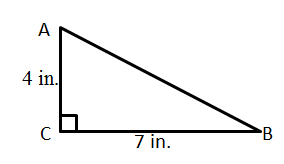1) Find the measure of angle A.
Step 1: Choose which trig ratio to use.

We need to determine how the two sides we know the length of are related to angle A. The 4 in. side is adjacent toA and the 7 in. side is oppositeA. The ratio that uses adjacent and opposite is the tangent.

Step 2: Substitute

write our trig ratio:Then, we substitute in the sides: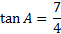Step 3: Solve

To solve for the angle, we must use
the inverse tangent or arctan.And the tan-1 button on your calculator
to find the answer. Round to the
nearest tenth.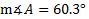2) Find the measure of angle B.

We could simply use the fact that the angles of a triangle add up to 180° to find the last angle. That would give us an answer of 29.7° . But once again, that assumes we found angle A correctly. The best thing to do will be to work through the steps for angle B. If we get 29.7° , then we will be fairly confident that we found both the correct answers.

Step 1: Choose which trig ratio to use
The 4 in. side is oppositeB and the 7 in. side is adjacent toB. The ratio that uses adjacent and opposite is once again the tangent.

Step 2: Substitute

Write our trig ratio: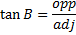Then, we substitute in the sides:Step 3: Solve

To solve for the angle, we must use
the inverse tangent or arctan.And the tan-1 button on your calculator
to find the answer. Round to the
nearest tenth.Remember, if your calculator doesn't seem to be giving you the right answer, read your manual or ask someone for help. Each calculator is different, and you have to enter the information in the correct order to get the right answer. Also, make sure your calculator is in Degree mode (not Radians)

Practice: Find the measure of the angles of these triangles:

1) mA    2) mB    3) mE    4) mF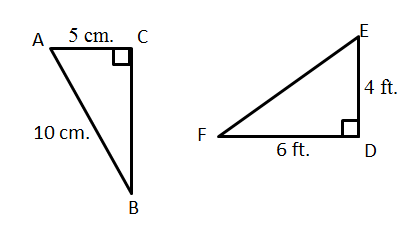Answers: 1) 60°  2) 30°  3) 56.3°  4) 33.7°